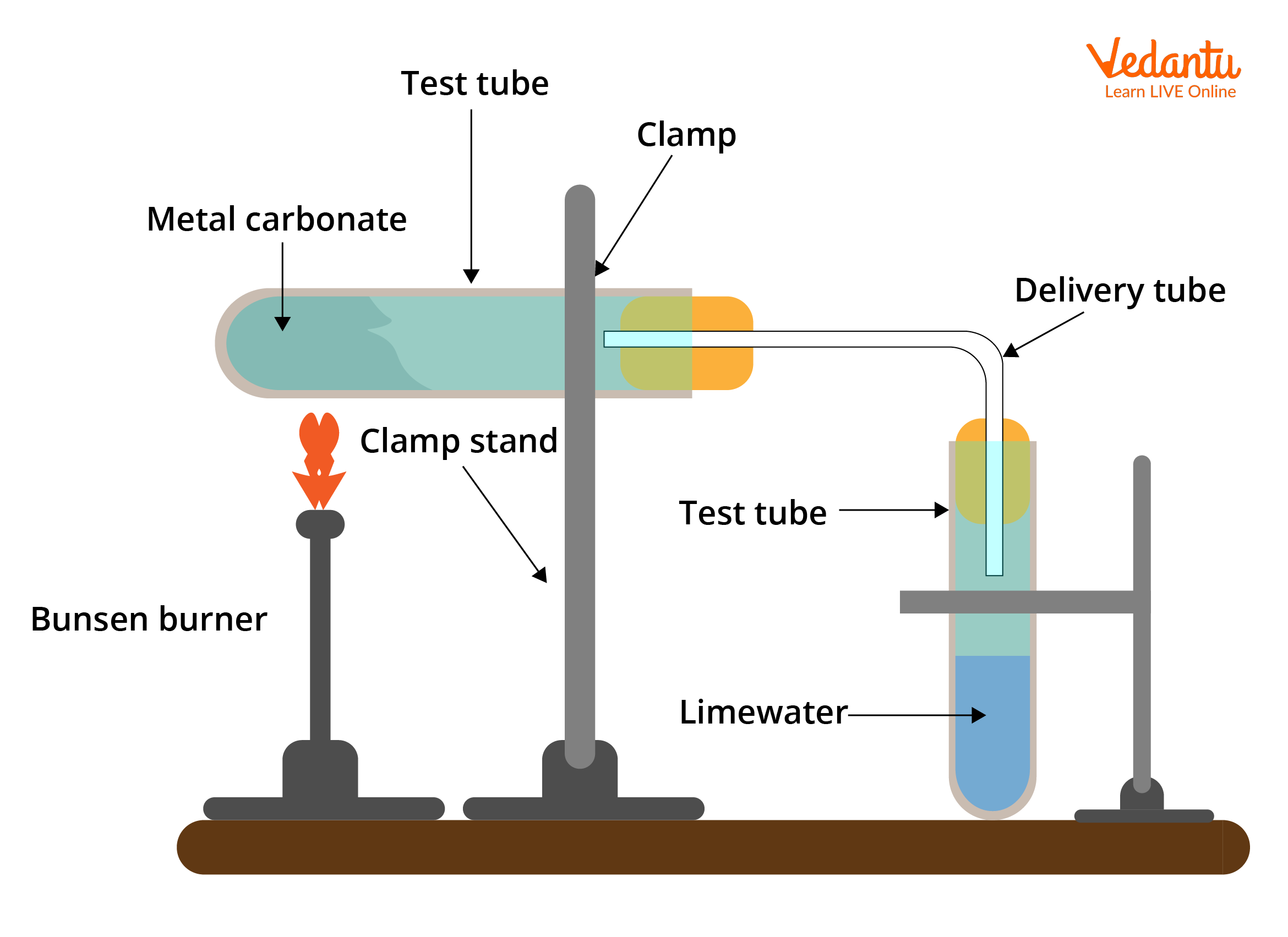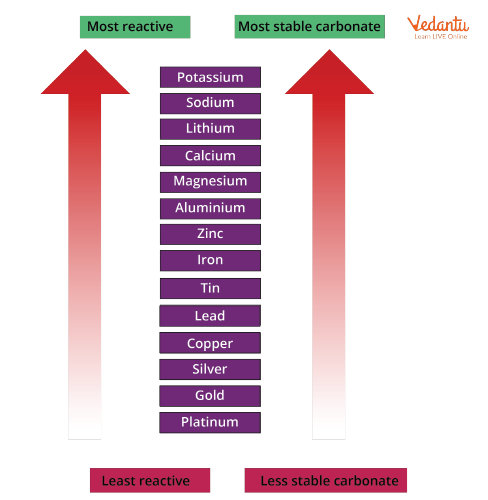# Thermal Stability of Salts for JEE## What is Thermal Decomposition Reaction?

The term "thermal" refers to the presence of heat. Breaking down is the process of decomposing. When a compound is heated and undergoes thermal breakdown, it is said to be thermal decomposition. At high temperatures, thermal breakdown reactions occur. Before breaking down into the products, the reactants need a lot of energy.

The reactant is the beginning chemical. It decomposes into simpler entities, which might be either elements or compounds. There is just one reactant in a thermal breakdown reaction, but two or more products. Thermal breakdown processes can occur in mercury oxide, copper carbonate, copper sulphate, copper hydroxide, sodium nitrate, lead carbonate, and zinc carbonate.

Endothermic reactions, such as thermal breakdown, are useful when cooking and baking cakes.

Thermal decomposition examples:

• Zinc carbonate on heating decomposes to zinc oxide and carbon dioxide by taking energy through heat.

$\mathrm{ZnCO}_{3} \xrightarrow{\Delta}\mathrm{ZnO}+\mathrm{CO}_{2}$

• When potassium chlorate is heated to a high temperature, it decomposes into potassium chloride and oxygen, and this reaction is utilised to prepare oxygen.

$2 \mathrm{KClO}_{3} \xrightarrow{\Delta} 2 \mathrm{KClO}+2 \mathrm{O}_{2}$

## Experimental Setup for Thermal Decomposition Reactions

### Apparatus used for these types of reactions includes:

• Test tube

• Delivery Tube

• Bunsen Burner

• Clamp StandExperimental Setup of Thermal Decomposition Reaction

When salts are heated in the test tube, they decompose into their respective compounds. The bunsen burner supplies heat to the test tube, which further decomposes to a gas and is supplied to the  delivery tube. When performing a thermal decomposition reaction, there is an important safety issue to keep in mind. It's essential to separate the test tube from the delivery tube after the reaction is complete. If this is not done, the gas in the delivery tube and test tube will contract as the apparatus cools. The cool contents will be sucked up from the delivery tube and into the extremely hot test tube, causing the glass to crack or even shatter. Suck-back is the term for this effect.

## Thermal Stability of Carbonates

By heating the metal carbonate and bubbling the carbon dioxide released by limewater, the stability of the metal carbonates can be determined in thermal decomposition of carbonates. Faster the limewater turns milky, faster the carbonate begins to decompose (meaning the carbonate is less stable).

The reactivity series of metals can be linked to the stability of metal carbonates. The more reactive a metal is, the more stable the metal carbonate becomes as it progresses in the series.Stability of Carbonates

## Thermal Stability of  Carbonates of Group 2

As we go down the group, the size of ions in the alkaline earth metal series increases. This leads to the bond strength increasing between metal and carbonates. The effect of ions on carbonate ions decreases. As the size of alkaline earth metal ions increases across the group, the effect of ions on carbonate ions decreases. The effect of ions on carbonate ions reduces as the size of alkaline earth metal ions increases across the group.

As a result, more heat is required to eliminate carbon dioxide and leave metal oxide.

Hence, stability of metal carbonates increases down the group in alkaline earth metals.

$\mathrm{BaCO}_{3}>\mathrm{SrCO}_{3}>\mathrm{CaCO}_{3}>\mathrm{MgCO}_{3}$

## Thermal Stability of Group 2 Nitrates

Metal oxide, nitrogen dioxide and oxygen gas are formed when group 2 nitrates are heated. When these compounds are heated, they produce white solids as well as brown nitrogen dioxide and oxygen gases. Magnesium and calcium nitrates crystallise with water in most cases, and the solid may dissolve in its own crystallisation water to form a white solution before decomposition begins.

General reaction for thermal decomposition of group 2 nitrates can be given as:

$2 \mathrm{M}\left(\mathrm{NO}_{3}\right)_{2} \xrightarrow{\Delta}2 \mathrm{MO}+4 \mathrm{NO}_{2}+\mathrm{O}_{2}$

The thermal stability of metal nitrate of group 2 also increases down the group.

$\mathrm{Ba}\left(\mathrm{NO}_{3}\right)_{2} > \mathrm{Sr}\left(\mathrm{NO}_{3}\right)_{2} > \mathrm{Ca}\left(\mathrm{NO}_{3}\right)_{2} > \mathrm{Mg}\left(\mathrm{NO}_{3}\right)_{2}$

## Thermal Stability of Group 2 Hydroxide

The metal hydroxides of group 2 decompose on heating, giving metal oxide and water. This is an example of thermal decomposition reaction:

$\mathrm{M}(\mathrm{OH})_{2} \rightarrow \mathrm{MO}+\mathrm{H}_{2} \mathrm{O}$

As we move down the group, the size of the element increases as the number of shells rises. The ionisation energy of an element reduces as its size increases, implying that it is easier for one element to transfer electrons to another, resulting in an increase in lattice enthalpy. The thermal stability of hydroxide is directly related to the lattice enthalpy value.

The thermal stability of hydroxide increases along the group in the case of alkaline earth metal.

$\mathrm{Ba}(\mathrm{OH})_{2} > \mathrm{Sr}(\mathrm{OH})_{2} > \mathrm{Ca}(\mathrm{OH})_{2} > \mathrm{Mg}(\mathrm{OH})_{2}$

## Thermal Dissociation Reaction

Reversible reactions are reactions that, once transformed into products, can readily be turned back into reactants. Thermal dissociation is one of these types of reversible reactions, since the products can be converted back to reactants.

Equilibrium is achieved in thermal dissociation according to the law of mass action, and can be described by either the equilibrium constant or the degree of dissociation, which is the ratio of dissociated molecules to total molecules. Thermal dissociation is frequently accompanied with heat absorption.

$2 \mathrm{H}_{2} \mathrm{O} \rightleftarrows 2 \mathrm{H}_{2}+\mathrm{O}_{2}$

## Conclusion

The term "thermal" refers to the presence of heat. Breaking down is the process of decomposing. When a compound is heated, it undergoes thermal breakdown and is said to be thermal decomposition. As we go down the group, the size of ions in the alkaline earth metal series increases. This leads to the bond strength increasing between metal and carbonates. The more reactive a metal is, the more stable the metal carbonate becomes as it progresses in the series.

The thermal stability of metal nitrate and hydroxides of group 2 also increases down the group. Thermal dissociation is one of these types of reversible reactions since the products can be converted back to reactants.

## FAQs on Thermal Stability of Salts for JEE

1. What is an electrolytic decomposition reaction?

An electrolytic breakdown reaction can occur when an electric current is transmitted through an aqueous solution of a compound. A good example of an electrolytic breakdown reaction is electrolysis of water. It can be used for a variety of purposes. In the industry, this reaction is used for preparation of aluminium, salt, chlorine and other. This reaction can be performed in an electrolytic cell. For example, water can be converted to H2 and O2 with a redox reaction.

The following reaction is involved:

$2 \mathrm{H}_{2} \mathrm{O} \rightarrow 2 \mathrm{H}_{2}+\mathrm{O}_{2}$

2. What is photo decomposition reaction?

A photo decomposition reaction is a type of decomposition reaction in which the reaction absorbs energy from photons and breaks down into its constituents. A source of photons is needed to perform such types of reaction. The breakdown of ozone into dioxygen and an oxygen radical, as shown in the chemical equation below, is an example of a photo decomposition reaction.

$\mathrm{O}_{3}+\mathrm{h} v \rightarrow \mathrm{O}_{2}+\mathrm{O}$

Photo decomposition reaction is also used in old photographic techniques. For example, silver halides breaks into silver metal and halogen gas in the presence of sun light.

Comment